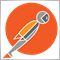# What does it mean by , bool x=(a,b); ?[Deleted]

What does it mean by , bool x=(a,b); ?

I am reading code . and i dont understand what's this about . a=true and b=false ?

```   if(CompareDoubles(sl,OrderStopLoss()) &&
CompareDoubles(tp,OrderTakeProfit()))return true;```

Thanks for answering2758

Tsz Ho Chow:

What does it mean by , bool x=(a,b); ?

I am reading code . and i dont understand what's this about . a=true and b=false ?

Thanks for answering

Compare Doubles implicitly returns true if the numbers are the same. How you should read this is if(true && true) return true